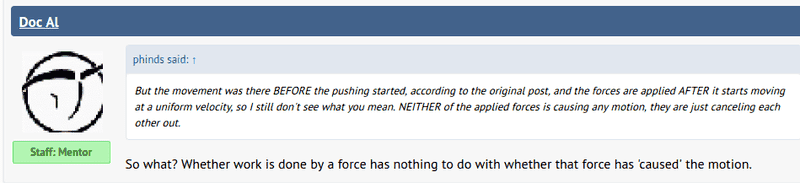# Does a force have to *cause* motion to do work?

I'm trying to understand the concept of work. I understand that no work can be done by a force if there is no displacement, but I have got confused about whether a force has to cause the displacement.

The site physicsclassroom.com says this:But a mentor on this site says this:This latter point seems reasonable to me since, if I raise a body with my hand then it is said that gravity has done negative work on that body; and gravity certainly hasn't caused the displacement.

Thank you.

A.T.
I'm trying to understand the concept of work. I understand that no work can be done by a force if there is no displacement, but I have got confused about whether a force has to cause the displacement.
The definition of work says nothing about "causation".

BiGyElLoWhAt
Gold Member
Yes it does. You have to have an acceleration for work to be done on an object. It's kinetic energy has to change (or some form of potential energy). If two equal forces act on a block in opposite direction whilst it is moving, they cancel each other out, and the velocity doesn't change. If the velocity doesn't change, no work was done on the block.
I suppose you could distinguish between no work and no net work, however, I don't see how that would be useful.

@A.T (he beat me)
It doesn't say it in the definition of work, but it can be deduced from CoE. ##E_i \pm \Delta W = E_f##
If ##E_i = E_f##, then it is quite clear that ##\Delta W = 0##

A.T.
If the velocity doesn't change, no work was done on the block.
You confuse "net work" and "work".

Dale
Mentor
2021 Award
I have got confused about whether a force has to cause the displacement.
I don't know how to identify which forces "caused" the displacement in general. I have never seen that requirement before, and don't know how to implement it.

BiGyElLoWhAt
Gold Member
No, I've just chosen to not distinguish between them. See the rest of my post:
I suppose you could distinguish between no work and no net work, however, I don't see how that would be useful.

BiGyElLoWhAt
Gold Member
Also, it's generally the integral of the net force that you're concerned with. If there is a non zero net force, there will be an acceleration.

Dale
Mentor
2021 Award
I suppose you could distinguish between no work and no net work, however, I don't see how that would be useful.
It is very useful. Take for example a person lifting a box by pulling on a massless rope at constant speed. The box gains gravitational PE, so the rope does work on the box, the person does work on the rope but the rope's PE does not change, the net work done on the rope is zero even though work is done both on and by the rope.

I don't see how the concept can be avoided in understanding any situation with energy transfer between 3 or more bodies.

BiGyElLoWhAt
Gold Member
I think I see what you're saying. I was thinking simply, with arbitrary forces acting on a single object.

A.T.
No, I've just chosen to not distinguish between them.
If the velocity doesn't change, no work was done on the block.
You can do net work on an object, without changing its velocity. For example by deforming it.

It is very useful. Take for example a person lifting a box by pulling on a massless rope at constant speed. The box gains gravitational PE...

Yes, this is the point that I've been wondering about. If a body is moving at a constant speed then there can be no net force acting upon it, and yet in this case it is gaining potential energy. Where is this energy coming from, given that the forces upwards and downwards are equal?

Thank you all for you responses by the way.

The confusion comes from counting gravity twice, in your analysis: both as a force and a potential.
Either represent gravity by a force and then you have zero net force and body moving with constant speed. The net work is zero and the body gains no energy.
Or you consider a body acted by one force moving in a gravitational potential. In this second case the work done by the non-gravitational force increases the potential energy.

jack action
Gold Member
Imagine someone pulls a block sliding on the ground at a constant velocity. The person's pulling force cancels the block's friction force. The person's pulling does work because of the displacement. The friction force does not do work, but it creates heat. The work done equals the heat released. The person's pulling force caused the motion.

If the person accelerates, the (increased) work done is still the (increased) pulling force times the displacement. The work done is now equal to the sum of the heat released by the friction and the gain in kinetic energy.

You don't need acceleration to do work, only a force and a displacement.

Same thing with your example: While lifting an object at constant velocity (i.e. lifting force is equal to the weight of the object), the lifting force does work and the weight does not. But the object has gain potential energy (i.e. it has the potential of doing the same amount work in reverse, ##mg\Delta h## = force X displacement). The work done equals the gain in potential energy. The lifting force is the one causing the motion.

Dale
Mentor
2021 Award
Where is this energy coming from, given that the forces upwards and downwards are equal?
From the rope. There is an energy flow from the man, to the rope, to the box, to the gravitational field.